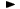ADVFN HomeHelpFinancialsKey FiguresNAV PS

# NAV PS

Net Assets Value per share ratio, is defined as Shareholders' funds attributable to equity interests divided by the number of shares in issue. Net assets is the total assets less the total liabilities i.e. the total shareholders funds plus preferences and minority interests. Figure is expressed in pence (currency being GBP) and the formula is the following:

= (total equity / shares in issue) * 100

Note: If company provides NAV per share (basic and diluted) figures in their Annual Report, the latest basic NAV per share figure is used.